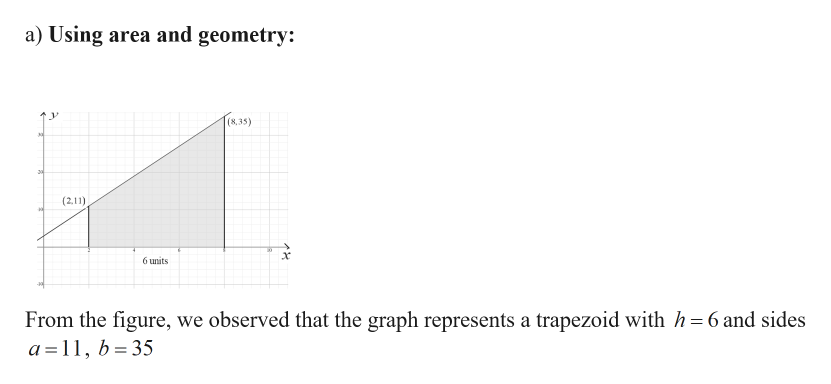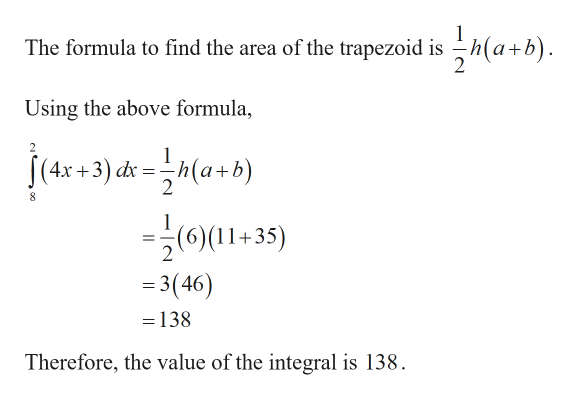# Evaluate integral 8 to 2 (4x + 3) dx in two ways:(a) Using area and geometry.(b) Using the Fundamental Theorem of Calculus.

Question
16 views

Evaluate integral 8 to 2 (4x + 3) dx in two ways:
(a) Using area and geometry.
(b) Using the Fundamental Theorem of Calculus.

check_circle

Step 1

Given:

Step 2

Calculation:help_outlineImage Transcriptionclosea) Using area and geometry: (8,35) (2.11) 6 units From the figure, we observed that the graph represents a trapezoid with h= 6 and sides a =11, b= 35 fullscreen
Step 3help_outlineImage TranscriptioncloseThe formula to find the area of the trapezoid is h(a+b). Using the above formula, +3) dk = -h(a+b) =(0)(11+35) = 3(46) =138 Therefore, the value of the integral is 138. fullscreen

### Want to see the full answer?

See Solution

#### Want to see this answer and more?

Solutions are written by subject experts who are available 24/7. Questions are typically answered within 1 hour.*

See Solution
*Response times may vary by subject and question.
Tagged in

### Other